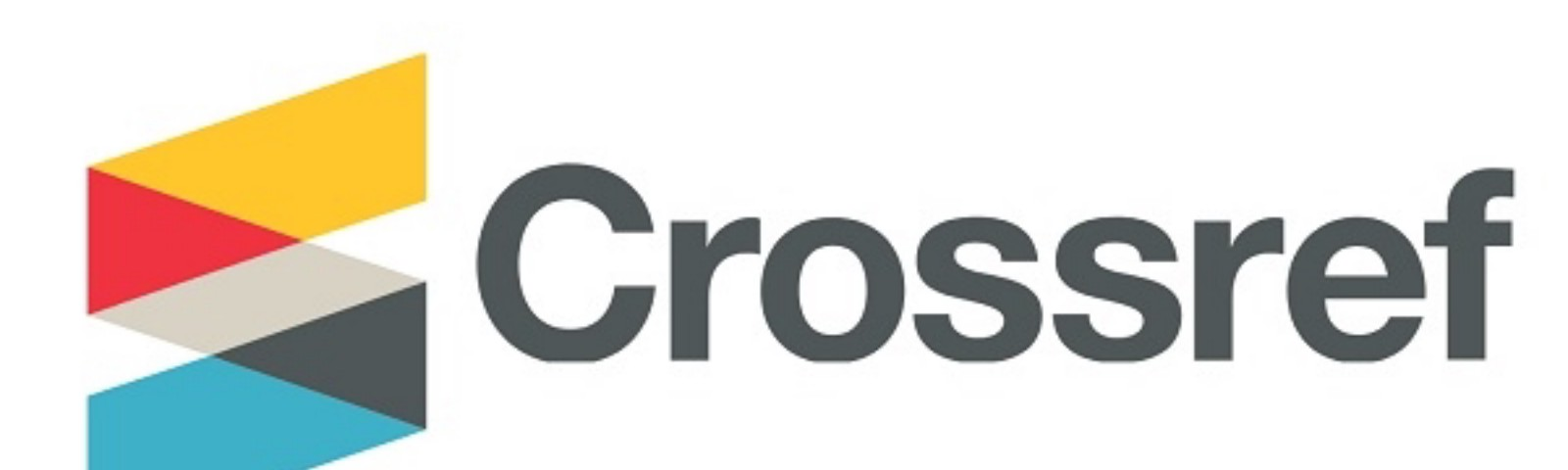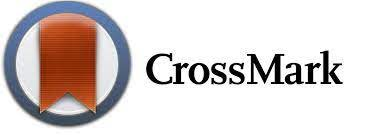- Quartile SJR 2021 = Q3
- Quartile CiteScore 2021 = Q3
- Quartile JCI 2020 = Q3
- SJR 2021 = 0.315
- CiteScore 2021 = 2.4
- SNIP 2021 = 0.6

- J. Citation Indicator (JCI 2020) = 0.24
- Acceptance Rate = 38%2.4
2021CiteScore

48th percentile
Powered byUser